# Laplace Transform

By on

Laplace transforms and their inverse are a mathematical technique which allows us to solve differential equations, by primarily using algebraic methods. This simplification in the solving of equations, coupled with the ability to directly implement electrical components in their transformed form, makes the use of Laplace transforms widespread in both electrical engineering and control systems engineering.

This note is a recap/review of Laplace theory and reference which can be used while carrying out day to day work.  It is not an introduction or tutorial and does assume some prior knowledge of the subject.

## Theory

The Laplace transform, named after Pierre-Simon Laplace who introduced the idea is defined as:

$F(s)=L{ f(t) }= ∫ 0 ∞ e −st f(t)dt$

where: S = σ + iω,  (σ and ω as real numbers)

the result is a function of s, and the transform can be written as:

$F(s)=L{ f(t) }$

Give the transform, the inverse can be found from:

$f(t)= L −1 { F(s) }= 1 2πi lim T→0 ∫ γ−iT γ+iT e st F(s) ds$

While we can use the above equations to find the Laplace transform (or it's inverse) for a given function, in practice the use of table is more common.  The following table lists the more common transforms and their inverse:

Time Function
f(t)
Laplace Transform
F(s)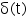, unit impulse 1, unit step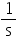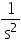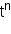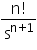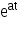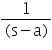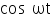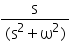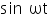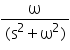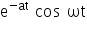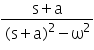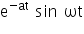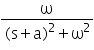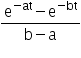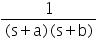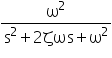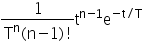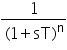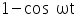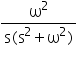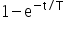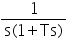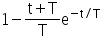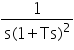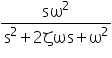### Useful Operations

Linearity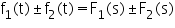Multiplication (by constant)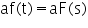Real Shift Theorem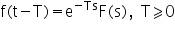Complex Shift Theorem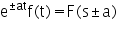Scaling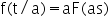Derivative (1st)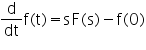Derivative (nth)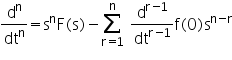Integral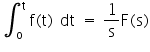Convolution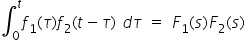Heaviside (step) Function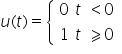### Partial Fractions

In finding the inverse transforms, it is helpful if the equation to be solved is expressed as a sum of fractions.  Partial fractions are a way to achieve this and the process is called resolving partial fractions.

To be able resolve partial fractions, the numerator needs to be of lesser degree than the denominator and depending on the type of partial fraction:

Linear factors in the denominator: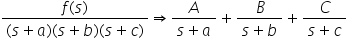Repeated linear factors in the denominator: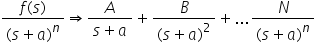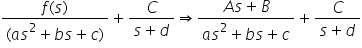Example: the following example illustrates how to use the method of partial fractions to resolve a simple equation using linear factors. Ffind the partial fractions of:

which can be resolved into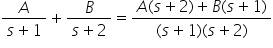From the above, it can be seen that: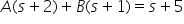By choosing values of s to make A or B equal to zero (s = -2, s = –1), the above can be solved to give A (=4) and B (=-3), giving us the final solution: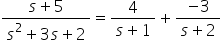## Application

One of the great things about Laplace Transforms is that the core electrical quantities (resistance, inductance and capacitance) can be easily represented in their Laplace form; simplifying the solving of circuits.  The table below summaries the time and Laplace representation of each quantity:

Time domain Laplace domain
v(t) i(t) F(s) V(S) I(S)

Resistor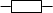R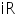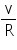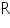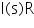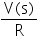InductorL
$L= di(t) dt$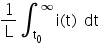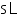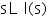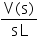Capacitor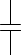C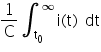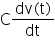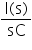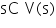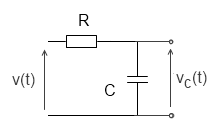Example:  consider a series circuit consisting of a resistor and capacitor as shown. A step voltage v(t) equal to V0 is applied at time zero.  What is the form of the voltage across the capacitor, vc(t).

To represent the input voltage, we can use the Heaviside (step):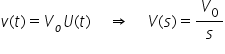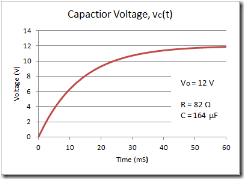From the above table, the Laplace transform of the current, i through the circuit can be written directly as: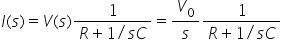and the Laplace voltage across capacitor as: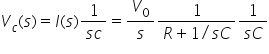Rearranging the equation and taking the inverse Laplace transforms, gives: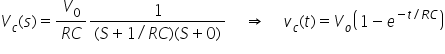More interesting Notes:Steven McFadyen

Steven has over twenty five years experience working on some of the largest construction projects. He has a deep technical understanding of electrical engineering and is keen to share this knowledge. About the author

What is a rectifier transformer?

I've recently come across this question a couple times browsing the internet. Decided to give a quick answer here. A rectifier transformer is a transformer...

Railway Electrification Voltages

This post is quick introduction and overview to different railway electrification voltages used in answer to a question sent in via email. While there...

Robotics - Home Innovations

We have a sister note to this (Robots - Interesting Video), in which I have posted some videos of interesting robots developed by commercial corporations...

RLC Circuit, Resistor Power Loss - some Modelica experiments

Modelica is an open source (free) software language for modelling complex systems. Having never used it before, I thought I would download a development...

Autonomous Vehicle Challenge

Two driverless and solar power vans have departed from Italy on their way to China via the silk road. During the 13,000 kM trip the vans will drive themselves...

Frame Leakage Protection

While not as popular as it once was, frame leakage protection does still have some use in some circumstances.  In essence frame leakage is an earth fault...

Low Voltage Fault Tables

The following tables provide quick order of magnitude fault levels for a a range of typical low voltage situations.

Battery Sizing

This article gives an introduction to IEEE 485 method for the selection and calculation of battery capacity.

Motor Efficiency Classification

Electric motors are one of the most widely used items of electrical equipment. Improving motor efficiency benefits include, reduced power demand, lower...

How to Calculate Motor Starting Time

Request to look at induction motor starting time have come up a few times on the site. Hopefully in this post, I give you guys some idea on how to calculate...## Have some knowledge to share

If you have some expert knowledge or experience, why not consider sharing this with our community.

By writing an electrical note, you will be educating our users and at the same time promoting your expertise within the engineering community.

To get started and understand our policy, you can read our How to Write an Electrical Note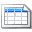Click here to view the notes list in a table formatClick here to view the notes indexed by tag